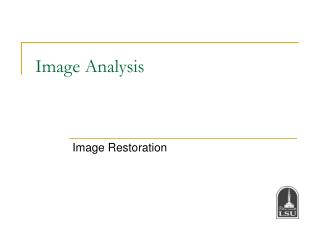Download PresentationImage Analysis

Image Analysis - PowerPoint PPT Presentation

Image Analysis. Image Restoration. Image Restoration. Image enhancement tries to improve subjective image quality. Image restoration tries to recover the original image. Noise Models. Noise may arise during image due to sensors, digitization, transmission, etc.I am the owner, or an agent authorized to act on behalf of the owner, of the copyrighted work described.
Download PresentationImage Analysis

Download Policy: Content on the Website is provided to you AS IS for your information and personal use and may not be sold / licensed / shared on other websites without getting consent from its author.While downloading, if for some reason you are not able to download a presentation, the publisher may have deleted the file from their server.

- - - - - - - - - - - - - - - - - - - - - - - - - - E N D - - - - - - - - - - - - - - - - - - - - - - - - - -
Presentation Transcript
1. Image Analysis Image Restoration

2. Image Restoration Image enhancement tries to improve subjective image quality. Image restoration tries to recover the original image. EE4780

3. Noise Models • Noise may arise during image due to sensors, digitization, transmission, etc. • Most of the time, it is assumed that noise is independent of spatial coordinates, and that there is no correlation between noise component and pixel value. • Noise may be considered as a random variable, its statistical behavior is characterized by a probability density function (PDF). Gaussian noise EE4780

4. Noise Models Uniform noise Impulse (salt-and-pepper) noise EE4780

5. Noise Models Original Noisy images and their histograms EE4780

6. Noise Models • How to estimate noise parameters? • If imaging device is available • Take a picture of a flat surface. • See the shape of the histogram; decide on the noise model. • Estimate the parameters. (e.g., find mean and standard deviation.) • When only images already generated are available • Get a small patch of image with constant gray level • Inspect histogram • Estimate the parameters EE4780

7. Restoration When There is Only Noise • Low-Pass Filters: • Smoothes local variations in an image. • Noise is reduced as a result of blurring. • For example, Arithmetic Mean Filter is  Convolve with a uniform filter of size m-by-n. EE4780

8. Restoration When There is Only Noise • Adaptive, local noise reduction filter • Let be the noise variance at (x,y). • Let be the local variance of pixels around (x,y). • Let be the local mean of pixels around (x,y). • We want a filter such that • If noise variance is zero, it should return g(x,y). • If local variance is high relative to noise variance, the filter should return a value close to g(x,y). (Therefore, edges are preserved!) • If two variances are equal, the filter should return the average of the pixels within the neighborhood. EE4780

9. Restoration When There is Only Noise • Median Filter • Replaces the value of a pixel by the median of intensities in the neighborhood of that pixel. • Is very effective against the salt-and-pepper noise. EE4780

10. Restoration When There is Only Noise • Adaptive Median Filter: The basic idea is to avoid extreme values • Let • z_min: minimum gray level value in a neigborhood of a pixel at (x,y). • z_max: maximum gray level value… • z_med: median… • z(x,y): gray level at (x,y). • Is z_med=z_min or z_med=z_max? (That is, is z_med an extreme value?) • No: • Is z(x,y) an extreme value? (Is z(x,y)=z_min or z(x,y)=z_max?) • No: Output is z(x,y) • Yes: Output is z_med. • Yes: Increase window size (to find a non-extreme z_med) and go to the first step. (When a maximum allowed window size is reached, stop and output z(x,y).) EE4780

11. Restoration When There is Only Noise Removing Periodic Noise with Band-Reject Filters Spikes are due to noise Periodic Noise Band-reject filter EE4780

12. Restoration When There is Only Noise Finding Periodic Noise from the Spectrum and Using Notch Filters Filter out these spikes Noise due to interference EE4780

13. Image Restoration Spatial domain: Frequency domain: EE4780

14. Image Restoration Inverse Filtering This could dominate signal. EE4780

15. Image Restoration EE4780

16. Image Restoration Cut off the inverse filter for large frequencies. (Signal-to-noise ratio is typically low for large frequencies.) EE4780

17. Image Restoration • Minimum Mean Square Error (Wiener) Filtering: • Find such that the expected value of error is minimized: • Solution is Investigate this equation for different signal-noise ratios. EE4780

18. Original image EE4780

19. Image Restoration EE4780

20. Least Squares Filtering Find F(u,v) that minimizes the following cost function: The solution is (Unconstrained solution) (See the derivations in the classroom) EE4780

21. Least Squares Filtering Find F(u,v) that minimizes the following cost function: Choose a P(u,v) to have a smooth solution. (A high-pass filter would do the trick.) EE4780

22. Least Squares Filtering The frequency domain solution to this optimization problem is (Constrained solution) where P(u,v) is the Fourier Transform of p(x,y), which is typically chosen as a high-pass filter. Example: EE4780

23. Least Squares Filtering EE4780